STOICHIOMETRY What is stoichiometry l Stoichiometry is the

• Slides: 29STOICHIOMETRYWhat is stoichiometry? l. Stoichiometry is the quantitative study of quantitative reactants and products in a chemical reaction. “stoichio” – Greek for element l “metry” = measurement l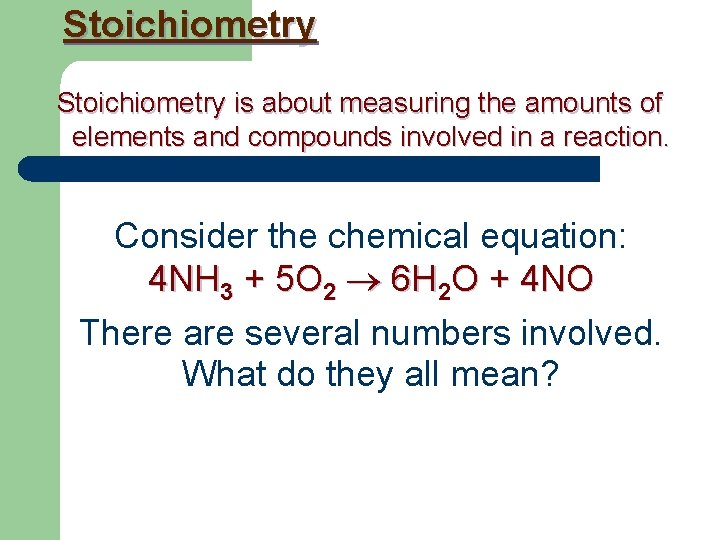Stoichiometry is about measuring the amounts of elements and compounds involved in a reaction. Consider the chemical equation: 4 NH 3 + 5 O 2 6 H 2 O + 4 NO There are several numbers involved. What do they all mean?Stoichiometry 4 NH 3 + 5 O 2 6 H 2 O + 4 NO Recall that Chemical formulas represent numbers of atoms NH 3 1 nitrogen and 3 hydrogen atoms O 2 2 oxygen atoms H 2 O 2 hydrogen atoms and 1 oxygen atom NO 1 nitrogen atom and 1 oxygen atom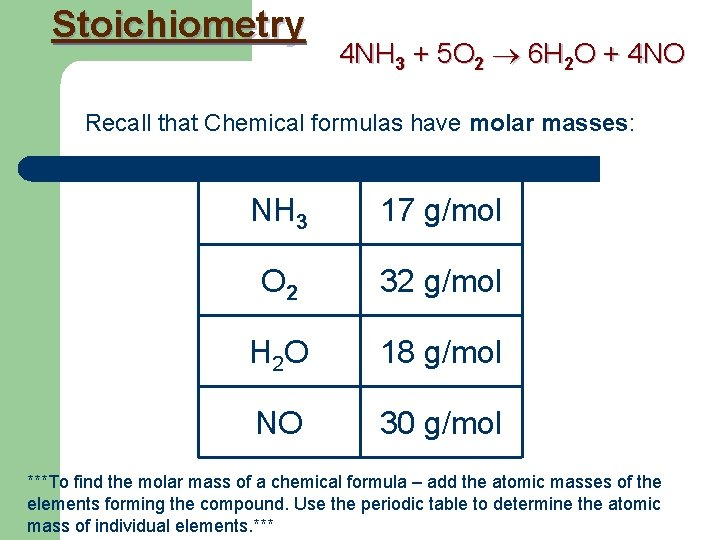Stoichiometry 4 NH 3 + 5 O 2 6 H 2 O + 4 NO Recall that Chemical formulas have molar masses: NH 3 17 g/mol O 2 32 g/mol H 2 O 18 g/mol NO 30 g/mol ***To find the molar mass of a chemical formula – add the atomic masses of the elements forming the compound. Use the periodic table to determine the atomic mass of individual elements. ***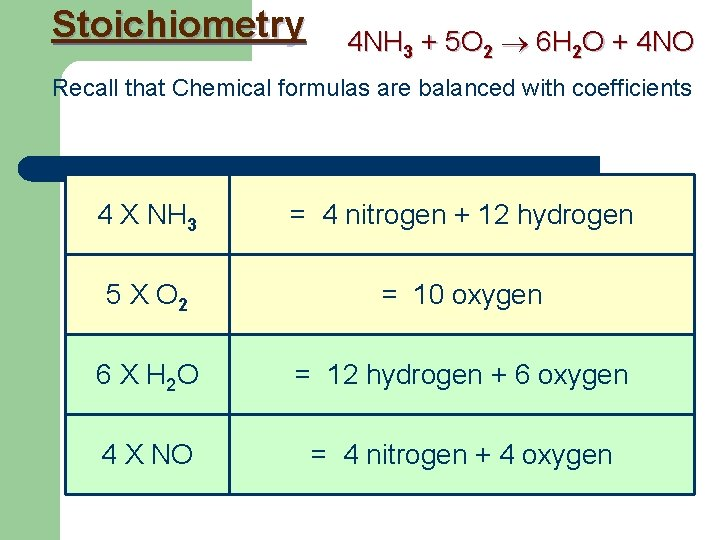Stoichiometry 4 NH 3 + 5 O 2 6 H 2 O + 4 NO Recall that Chemical formulas are balanced with coefficients 4 X NH 3 = 4 nitrogen + 12 hydrogen 5 X O 2 = 10 oxygen 6 X H 2 O = 12 hydrogen + 6 oxygen 4 X NO = 4 nitrogen + 4 oxygenStoichiometry 4 NH 3 + 5 O 2 6 H 2 O + 4 NO With Stoichiometry we find out that 4 : 5 : 6 : 4 do more than just multiply atoms. 4 : 5 : 6 : 4 Are what we call a mole ratio.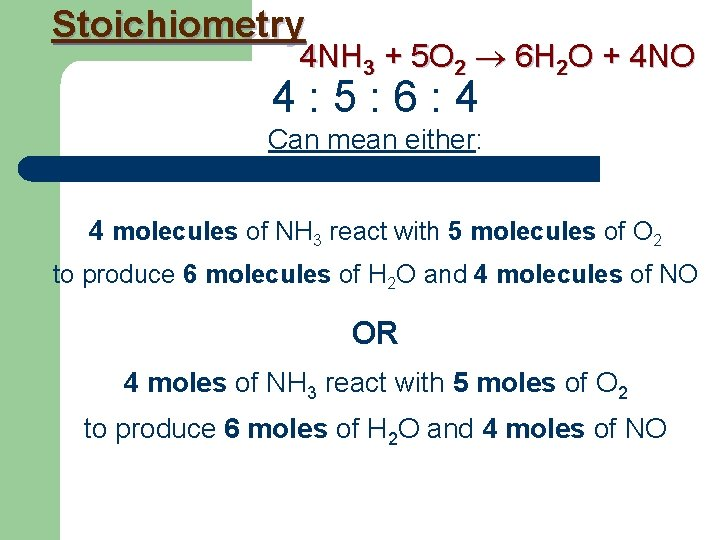Stoichiometry 4 NH 3 + 5 O 2 6 H 2 O + 4 NO 4 : 5 : 6 : 4 Can mean either: 4 molecules of NH 3 react with 5 molecules of O 2 to produce 6 molecules of H 2 O and 4 molecules of NO OR 4 moles of NH 3 react with 5 moles of O 2 to produce 6 moles of H 2 O and 4 moles of NO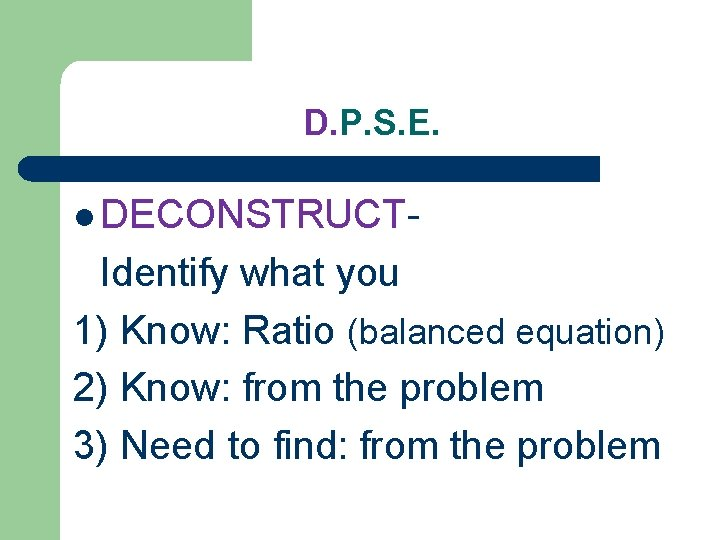D. P. S. E. l DECONSTRUCT- Identify what you 1) Know: Ratio (balanced equation) 2) Know: from the problem 3) Need to find: from the problemD. P. S. E. l Plan- Decide what type of pathway or equation you need to use. l We are going to start with using ratios, equalities, cross-multiplying and then dividing as needed (as we did last semester)D. P. S. E. l Solve – Insert numbers with units in proper places in your PlanD. P. S. E. l Evaluate – Circle your answer with units, and check it to see if it makes sense. l Is it too big, too small, does it make logical sense?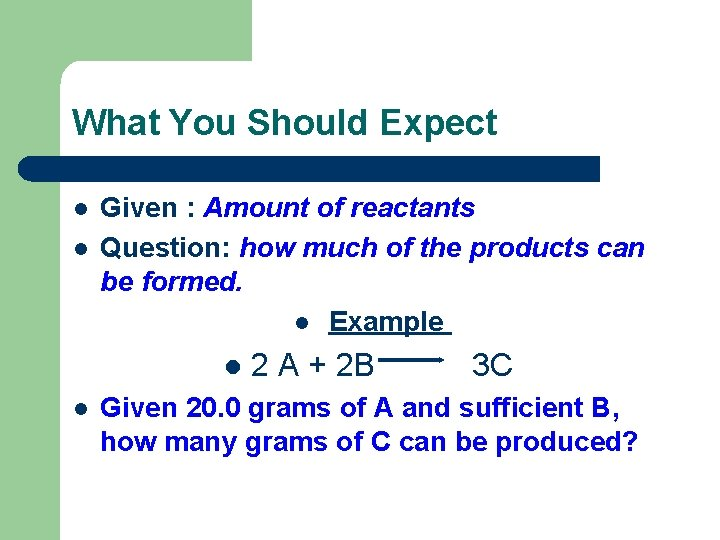What You Should Expect l l Given : Amount of reactants Question: how much of the products can be formed. l Example l l 2 A + 2 B 3 C Given 20. 0 grams of A and sufficient B, how many grams of C can be produced?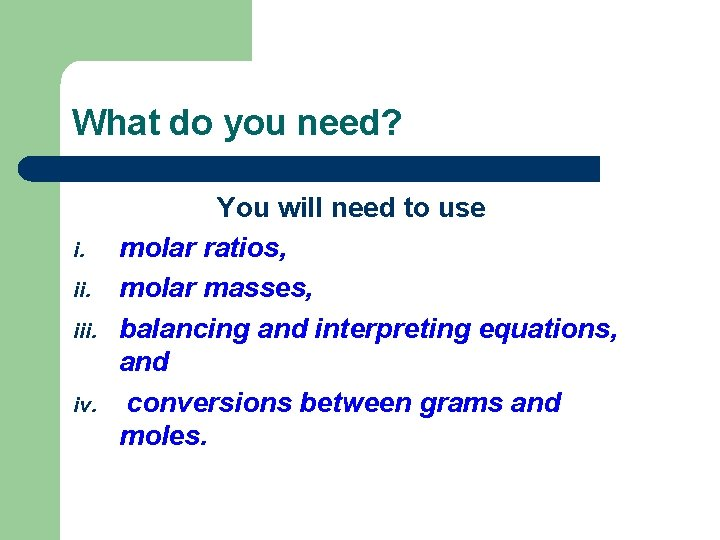What do you need? i. iii. iv. You will need to use molar ratios, molar masses, balancing and interpreting equations, and conversions between grams and moles.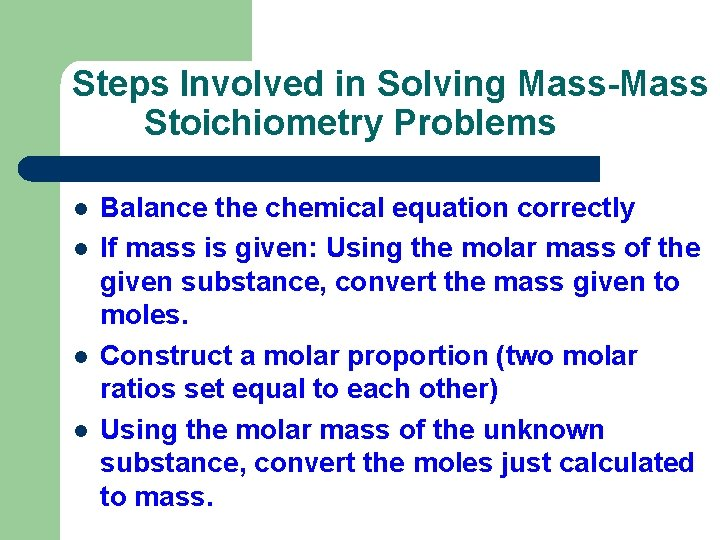Steps Involved in Solving Mass-Mass Stoichiometry Problems l l Balance the chemical equation correctly If mass is given: Using the molar mass of the given substance, convert the mass given to moles. Construct a molar proportion (two molar ratios set equal to each other) Using the molar mass of the unknown substance, convert the moles just calculated to mass.Mole Ratios A mole ratio converts moles of one compound in a balanced chemical equation into moles of another compound.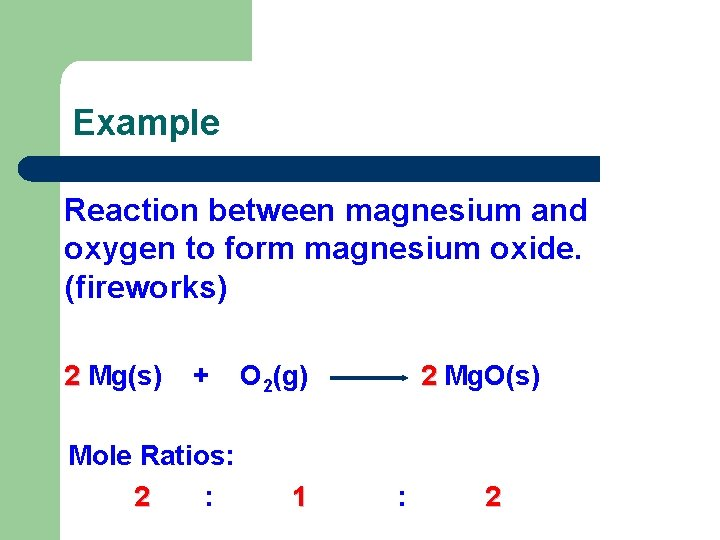Example Reaction between magnesium and oxygen to form magnesium oxide. (fireworks) 2 Mg(s) + Mole Ratios: 2 : O 2(g) 1 2 Mg. O(s) : 2Practice Problems 1) N 2 + 3 H 2 ---> 2 NH 3 Write the mole ratios for N 2 to H 2 and NH 3 to H 2. 2) A can of butane lighter fluid contains 1. 20 moles of butane (C 4 H 10). Calculate the number of moles of carbon dioxide given off when this butane is burned.Mole-Mole Problems Using the practice question 2) from the previous slide: Equation of reaction 2 C 4 H 10 + 13 O 2 8 CO 2 + 10 H 2 O Mole ratio C 4 H 10 CO 2 Know from bal eq: 2 : 8 [ coefficients] Simplify if needed: 1 : 4 What to Find: 1. 2 : X [ problem] By cross-multiplication: (4)(1. 2) = (1)(x) X = 4. 8 mols of CO 2 given off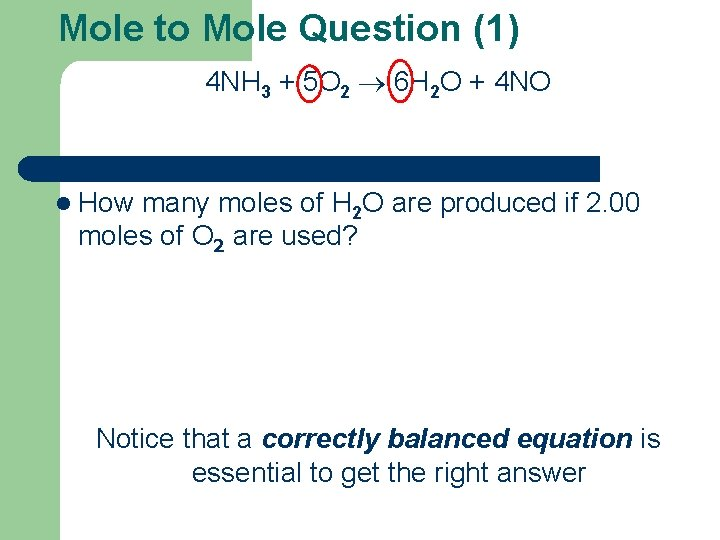Mole to Mole Question (1) 4 NH 3 + 5 O 2 6 H 2 O + 4 NO l How many moles of H 2 O are produced if 2. 00 moles of O 2 are used? Notice that a correctly balanced equation is essential to get the right answer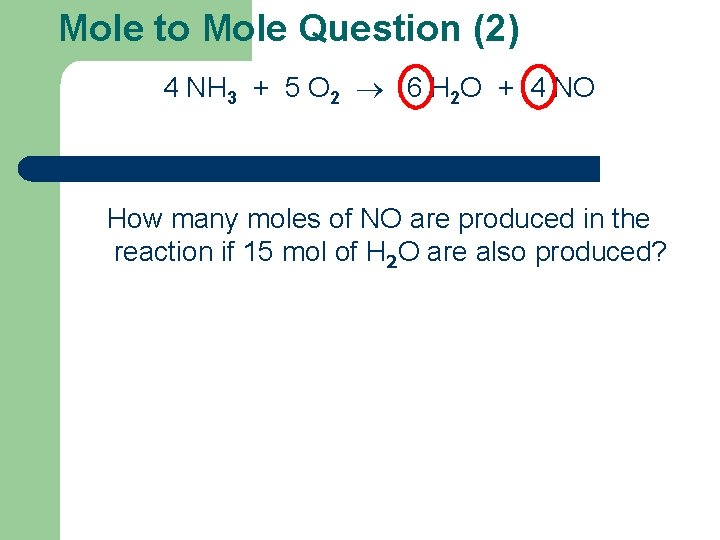Mole to Mole Question (2) 4 NH 3 + 5 O 2 6 H 2 O + 4 NO How many moles of NO are produced in the reaction if 15 mol of H 2 O are also produced?Mole-Mass Problems Make sure you have molar masses worked out for all compounds l Set up for grid making sure to use units correctly l Cross-multiply and isolate your variable (be sure to recognize those units that cancel out) lExample for Mole-Mass Problems l Problem 1: 1. 50 mol of KCl. O 3 decomposes. How many grams of O 2 will be produced? [K = 39, Cl = 35. 5, O = 16] 2 KCl. O 3 2 KCl + 3 O 2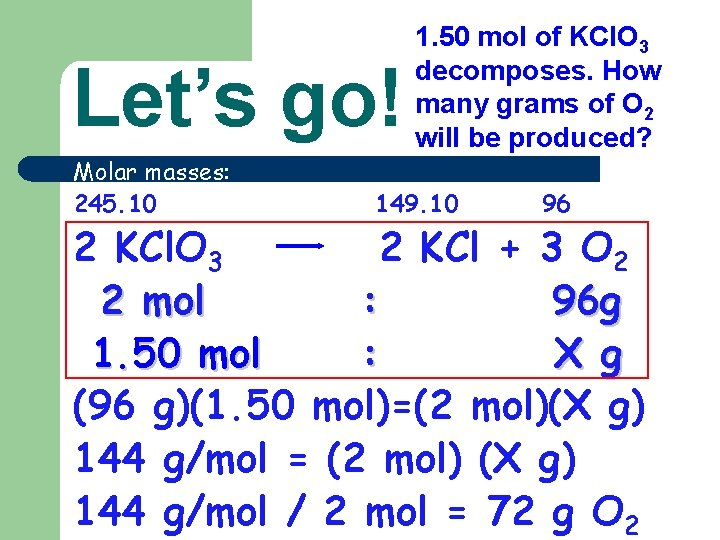Let’s go! Molar masses: 245. 10 1. 50 mol of KCl. O 3 decomposes. How many grams of O 2 will be produced? 149. 10 96 2 KCl. O 3 2 KCl + 3 O 2 2 mol : 96 g 1. 50 mol : X g (96 g)(1. 50 mol)=(2 mol)(X g) 144 g/mol = (2 mol) (X g) 144 g/mol / 2 mol = 72 g O 2Try This: We want to produce 2. 75 mol of KCl. How many grams of KCl. O 3 would be required? Soln l KCl. O 3 KCl : 245. 10 g : 2 mol X g : 2. 75 mol (2 mol)(X g) = (2. 75 mol)(245. 10 g) (2 mol)(X g) = 674. 025 g/mol X = 337. 01 grams of KCl. O 3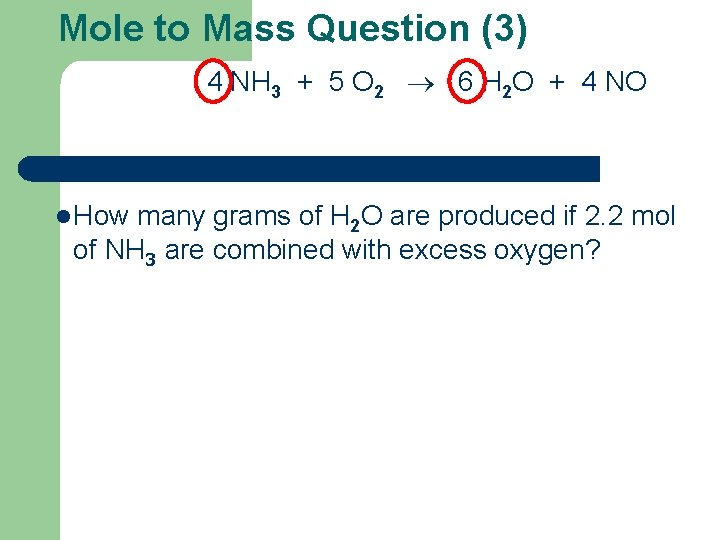Mole to Mass Question (3) 4 NH 3 + 5 O 2 6 H 2 O + 4 NO l How many grams of H 2 O are produced if 2. 2 mol of NH 3 are combined with excess oxygen?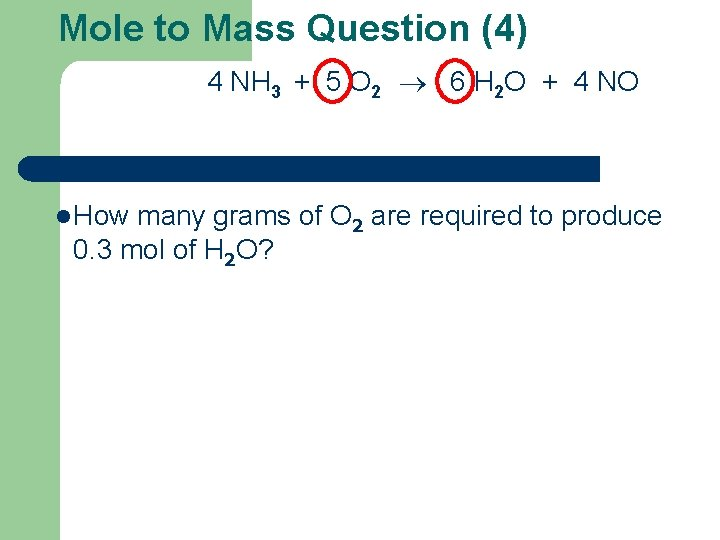Mole to Mass Question (4) 4 NH 3 + 5 O 2 6 H 2 O + 4 NO l How many grams of O 2 are required to produce 0. 3 mol of H 2 O?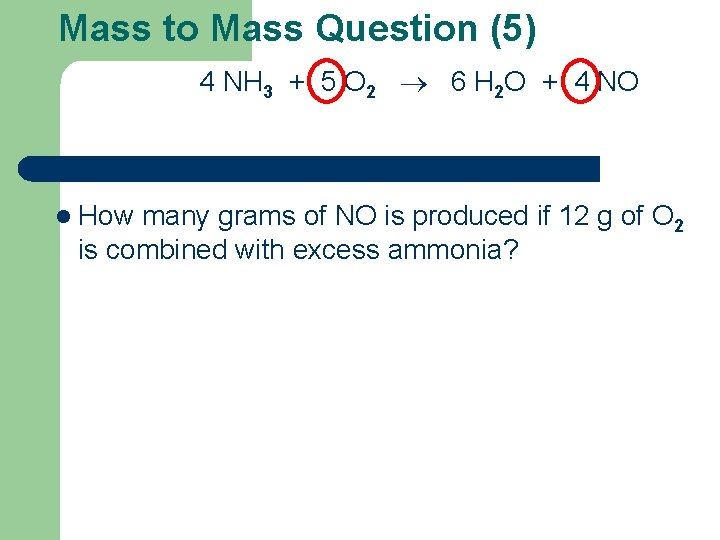Mass to Mass Question (5) 4 NH 3 + 5 O 2 6 H 2 O + 4 NO l How many grams of NO is produced if 12 g of O 2 is combined with excess ammonia?Have we learned it yet? Try these on your own - 4 NH 3 + 5 O 2 6 H 2 O + 4 NO a) How many moles of H 2 O can be made using 1. 6 mol NH 3? b) What mass of NH 3 is needed to make 0. 75 mol NO? c) How many grams of NO can be made from 47 g of NH 3?